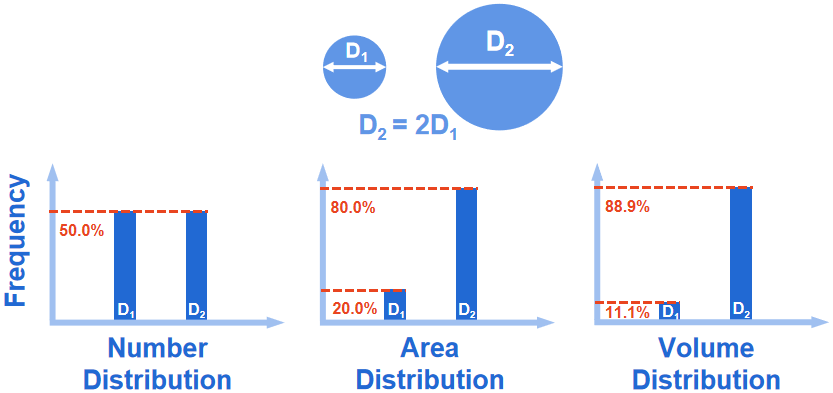Hmoe > Learn > Wiki > What are the differences between different distribution types?

# What are the differences between different distribution types?

2023-07-12WIKI

The particle size distribution can be displayed in 3 different ways called distribution types. The most commonly used distribution type in laser diffraction particle size analysis is the volume distribution, as the volume is what is measured. The measurement technology restricts the distribution type used. For example, image analysis, in which the particle projections are identified, and the projected areas are calculated, resulting in area distribution, while the signal received by the laser diffraction particle size analyzer reflects the volume of the particle. When the distribution types are different, the results vary greatly. Suppose there are two particles, and these two particles are present in equal numbers. Their diameters are D1 = 1μm and D2 = 2μm, which is a 2 to 1 diameter ratio, then depending on which distribution type is used, the results will vary, as shown below.As the diameter ratio increases from 2 to 1, to 3 to 1, the number distribution will be constant at 50%/50%, whereas the percentage of D1 will dramatically reduce for both the area and volume distributions.Struggling to grasp particle analysis concepts? Our new Beginner's Guide to Particle Analysis is here to help you grasp these concepts in the most accessible manner possible. Download it as a PDF to read on the train, on the plane, or wherever else there’s no internet.